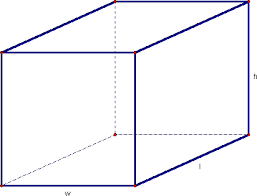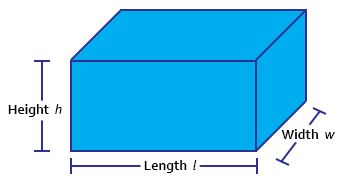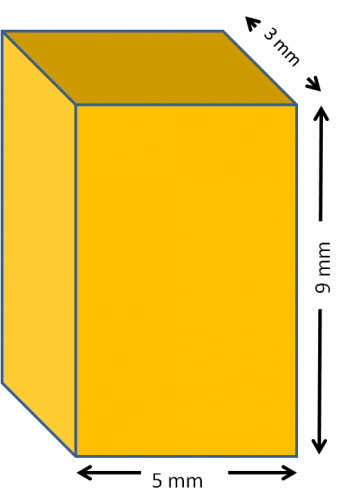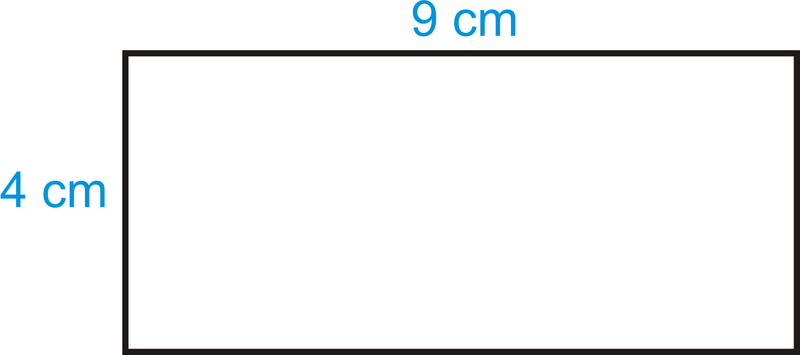## How to Calculate and Solve for the Width, Height, Length and Area of a Rectangular Prism | The Calculator EncyclopediaThe image above is a rectangular prism.

To compute the area of a rectangular prism requires three essential parameters which are the length, width and height of the rectangular prism.

A = 2wl + 2hl + 2hw

Where;
A = Area of the rectangular prism
l = Length of the rectangular prism
w = Width of the rectangular prism
h = Height of the rectangular prism

Let’s solve an example;
Find the area of a rectangular prism with a width of 10 cm, a height of 17 cm and a length of 14 cm.

This implies that;
l = Length of the rectangular prism = 14 cm
w = Width of the rectangular prism = 10 cm
h = Height of the rectangular prism = 17 cm

A = 2wl + 2hl + 2hw
A = 2 x 10 x 14 + 2 x 17 x 14 + 2 x 17 x 10
A = 280 + 476 + 340
A = 1096

Therefore, the area of the rectangular prism is 1096 cm2.

Calculating the Length of a Rectangular Prism using the Area, Width and Height of the Rectangular Prism.

l = A – 2hw / 2 (w + h)

Where;
l = Length of the rectangular prism
A = Area of the rectangular prism
w = Width of the rectangular prism
h = Height of the rectangular prism

Let’s solve an example;
Find the length of a rectangular prism with a width of 9 cm, a height of 12 cm and a Area of 250 cm2.

This implies that;
w = Width of the rectangular prism = 9 cm
h = Height of the rectangular prism = 12 cm
A = Area of the rectangular prism = 250 cm2

l = A – 2hw / 2 (w + h)
l = 250 – 2 x 12 x 9 / 2 (9 + 12)
l = 250 – 216 / 2 (21)
l = 34 / 42
l = 0.81

Therefore, the length of the rectangular prism is 0.81 cm.

## How to Calculate and Solve for the Width, Height, Length and Volume of a Rectangular Prism | Nickzom CalculatorThe image above is a rectangular prism.

To compute the volume of a rectangular prism requires three essential parameters which are the length, width and height of the rectangular prism.

V = lwh

Where;
V = Volume of the rectangular prism
l = Length of the rectangular prism
w = Width of the rectangular prism
h = Height of the rectangular prism

Let’s solve an example;
Find the volume of a rectangular prism with a length of 18 cm, a width of 12 cm and a height of 21 cm.

This implies that;
l = Length of the rectangular prism = 18 cm
w = Width of the rectangular prism = 12 cm
h = Height of the rectangular prism = 21 cm

V = lwh
V = 18 x 12 x 21
V = 4536

Therefore, the volume of the rectangular prism is 4536 cm3.

Calculating the Length of a Rectangular Prism using the Volume, Width and Height of the Rectangular Prism.

l = V / wh

Where;
l = Length of the rectangular prism
V = Volume of the rectangular prism
w = Width of the rectangular prism
h = Height of the rectangular prism

Let’s solve an example;
Find the length of a rectangular prism with a width of 19 cm, a height of 24 cm and a Volume of 250 cm3.

This implies that;
w = Width of the rectangular prism = 19 cm
h = Height of the rectangular prism = 24 cm
V = Volume of the rectangular prism = 250 cm3

l = V / wh
l = 250 / 19 x 24
l = 250 / 456
l = 0.55

Therefore, the length of the rectangular prism is 0.55 cm.

## How to Calculate the Volume, Length, Width and Height of a Cuboid | Nickzom CalculatorThe image above represents a cuboid.
To compute the volume of a cuboid requires three essential parameters which are the length, width and height of the cuboid.

The formula for computing the volume of a cuboid is:

V = lwh

Where:
V = Volume of the Cuboid
l = Length of the Cuboid
w = Width of the Cuboid
h = Height of the Cuboid

Let’s solve an example
Find the volume of a cuboid with a length of 6 cm, width of 2 cm and a height of 10 cm.

This implies that:
l = length of the cuboid = 6
w = width of the cuboid = 2
h= = height of the cuboid = 10

V = lwh
V = 6 x 2 x 10
V = 120

Therefore, the volume of the cuboid is 120 cm3.

Calculating the Length of a cuboid when Volume, Width and Height are Given

The formula is l = V / (w)(h)

Where;
V = Volume of the Cuboid
l = Length of the Cuboid
w = Width of the Cuboid
h = Height of the Cuboid

Let’s solve an example:
Find the length of a cuboid with a volume of 440 cm3 , a width of 5 cm and a height of 11 cm.

This implies that;
V = Volume of the cuboid = 440 cm3
w = width of the cuboid = 5 cm
h  = height of the cuboid = 11 cm

l =  V / (w)(h)
l = 440 / (5)(11)
l = 440 / 55
l = 8 cm

Therefore, the length of the cuboid is 8 cm.

Calculating the Width of a cuboid when Volume, Length and Height are Given

The formula is w = V / (l)(h)

Where;
V = Volume of the Cuboid
l = Length of the Cuboid
w = Width of the Cuboid
h = Height of the Cuboid

Let’s solve an example:
Find the width of a cuboid with a volume of  180 cm3 , a length of 6 cm and a height of 10 cm

This implies that;
V = Volume of the cuboid = 180 cm3
l = length of the cuboid = 6 cm
h  = height of the cuboid = 10 cm

w =  V / (l)(h)
w = 180 / (6)(10)
w = 180 / 60
w = 3 cm

Therefore, the width of the cuboid is 3 cm.

Calculating the Height of a cuboid when Volume, Length and Width are Given

The formula is h = V / (l)(w)

Where;
V = Volume of the Cuboid
l = Length of the Cuboid
w = Width of the Cuboid
h = Height of the Cuboid

Let’s solve an example:
Find the height of a cuboid with a volume of 195 cm3 , a length of 5 cm and a width of 3 cm

This implies that;
V = Volume of the cuboid = 195 cm3
l = length of the cuboid = 5 cm
w  = width of the cuboid = 3 cm

h =  V / (l)(w)
h = 195 / (5)(3)
h = 195 / 15
h = 13 cm

Therefore, the height of the cuboid is 13 cm.

## How to Calculate and Solve for the Area, Length, Width and Height of a Cuboid | Nickzom CalculatorThe image above represents a cuboid.
To compute the area of a cuboid requires three essential parameters which are the length, width and height of the cuboid.

The formula for computing the area of a cuboid is:

A = 2(lw + lh + wh)

Where:
A = Area of the Cuboid
l = Length of the Cuboid
w = Width of the Cuboid
h = Height of the Cuboid

Let’s solve an example
Find the area of a cuboid with a length of 5 cm, width of 3 cm and a height of 9 cm.

This implies that:
l = length of the cuboid = 5
w = width of the cuboid = 3
h= = height of the cuboid = 9

A = 2(lw + lh + wh)
A = 2(5 x 3 + 5 x 9 + 3 x 9)
A = 2(87)
A = 174

Therefore, the area of the cuboid is 174 cm2.

Calculating the Length of a cuboid when Area, Width and Height are Given

The formula is l = A – 2(w)(h) / 2(w) + 2(h)

Where;
A = Area of the Cuboid
l = Length of the Cuboid
w = Width of the Cuboid
h = Height of the Cuboid

Let’s solve an example:
Find the length of a cuboid with an area of 140 cm2 , a width of 4 cm and a height of 12 cm

This implies that;
A = Area of the cuboid = 140 cm2
w = width of the cuboid = 4 cm
h  = height of the cuboid = 12 cm

l =  A – 2(w)(h) / 2(w) + 2(h)
l = 140 – 2(4)(12) / 2(4) + 2(12)
l = 140 – 96 / 8 + 24
l = 44 / 32
l = 1.375 cm

Therefore, the length of the cuboid is 1.375 cm.

Calculating the Width of a cuboid when Area, Length and Height are Given

The formula is w = A – 2(l)(h) / 2(l) + 2(h)

Where;
A = Area of the Cuboid
l = Length of the Cuboid
w = Width of the Cuboid
h = Height of the Cuboid

Let’s solve an example:
Find the width of a cuboid with an area of 200 cm2 , a length of 5 cm and a height of 12 cm

This implies that;
A = Area of the cuboid = 200 cm2
l = length of the cuboid = 5 cm
h  = height of the cuboid = 12 cm

w =  A – 2(l)(h) / 2(l) + 2(h)
w = 200 – 2(5)(12) / 2(5) + 2(12)
w = 200 – 120 / 10 + 24
w = 80 / 34
w = 2.353 cm

Therefore, the width of the cuboid is 2.353 cm.

Calculating the Height of a cuboid when Area, Length and Width are Given

The formula is h = A – 2(l)(w) / 2(l) + 2(w)

Where;
A = Area of the Cuboid
l = Length of the Cuboid
w = Width of the Cuboid
h = Height of the Cuboid

Let’s solve an example:
Find the height of a cuboid with an area of 300 cm2 , a length of 6 cm and a width of 2 cm

This implies that;
A = Area of the cuboid = 300 cm2
l = length of the cuboid = 6 cm
w  = width of the cuboid = 2 cm

h =  A – 2(l)(w) / 2(l) + 2(w)
h = 300 – 2(6)(2) / 2(6) + 2(2)
h = 300 – 24 / 12 + 4
h = 276 / 16
h = 17.25 cm

Therefore, the height of the cuboid is 17.25 cm.

## How to Calculate and Solve for the Perimeter, Length and Width of a Rectangle | The Calculator EncyclopediaThe image above is a rectangle with a length of 8 cm and a width of 5 cm.
To compute the perimeter of a rectangle requires two parameters which are the length of the rectangle and the width of the rectangle.

The formula for calculating the perimeter of a rectangle is:

P = 2 (l + w)

Where:

P = Perimeter of a Rectangle
l = Length of a Rectangle
w = Width of a Rectangle

Let’s solve an example:
Find the perimeter of a rectangle where the length of the rectangle is 8 cm and the width of the rectangle is 5 cm.

This implies that:
l = Length of the Rectangle = 8 cm
w = Width of the Rectangle = 5 cm

P = 2 (l + w)
P = 2 (8 + 5)
P = 2 (13)
P = 26

Therefore, the perimeter of the rectangle is 26 cm.

How to Calculate the Length of a Rectangle when the Perimeter of the Rectangle and the Width of the Rectangle is Given

l = P / 2 – w

Where:
l = Length of the Rectangle
P = Perimeter of the Rectangle
w = Width of the Rectangle

## How to Calculate and Solve for the Area, Width and Length of a Rectangle | The Calculator EncyclopediaThe image above is a rectangle with a length of 9 cm and a width of 4 cm.
To compute the area of a rectangle requires two essential parameters which are the length of the rectangle and the width of the rectangle.

The formula for calculating the area of a rectangle is:

A = lw

Where:

A = Area of a Rectangle
l = Length of a Rectangle
w = Width of a Rectangle

Let’s solve an example
Find the area of a rectangle where the length of the rectangle is 9 cm and the width of the rectangle is 4 cm.

This implies that:
l = Length of the Rectangle = 9 cm
w = Width of the Rectangle = 4 cm

A = lw
A = (9)(4)
A = 36

Therefore, the area of the rectangle is 36 cm2.

How to Calculate the Length of a Rectangle when the Area and Width of the Rectangle is Given

The formula for calculating the length of the rectangle is:

l = A / w

Where:

l = Length of the Rectangle
A = Area of the Rectangle
w = Width of the Rectangle

Let’s solve an example:
Find the length of a rectangle where the area of the rectangle is 45 cm2 and the width of the rectangle is 9 cm.

This implies that:
A = Area of the Rectangle = 45 cm2
w = Width of the Rectangle = 9 cm

l = A / w
l = 45 / 9
l = 5

Therefore, the length of the rectangle is 5 cm.

How to Calculate the Width of a Rectangle when the Area and Length of the Rectangle is Given

The formula for calculating the width of the rectangle is:

w = A / l

Where:

w = Width of the Rectangle
A = Area of the Rectangle
l = Length of the Rectangle